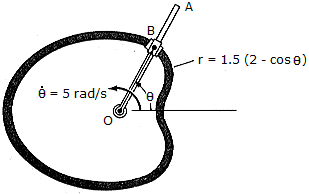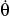# Engineering Mechanics - KOP: Force and Acceleration - Discussion

### Discussion :: KOP: Force and Acceleration - General Questions (Q.No.1)

1.Rod OA rotates counterclockwise with a constant angular rate of= 5 rad/s. The double collar B is pin-connected together such that one collar slides over the rotating rod and the other slides over the horizontal curved rod, of which the shape is a limacon described by the equation r - 1.5(2 - cos) ft. If both collars weigh 0.75 lb, determine the normal force which the curved path exerts on one of the collars, and the force that OA exerts on the other collar at the instant= 90°.

 [A]. FOA = 0.873 lb, Fcurve = 1.953 lb [B]. FOA = 0, Fcurve = 1.953 lb [C]. FOA = 1.747 lb, Fcurve = 0 [D]. FOA = 2.87 lb, Fcurve = 6.41 lb

Explanation:

No answer description available for this question.